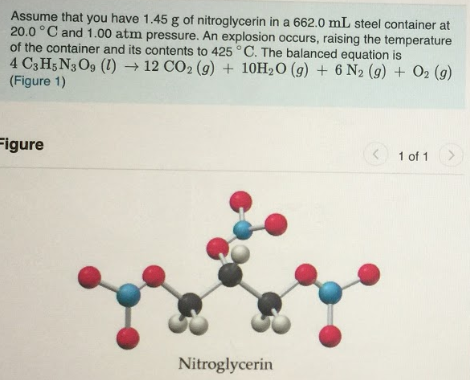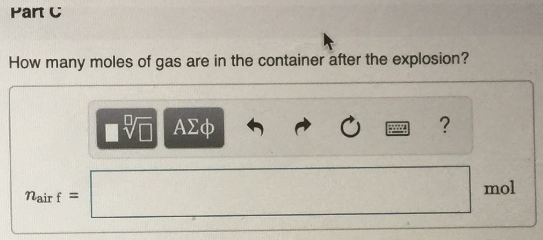# Assume that you have 1.45 g of nitroglycerin in a 662.0 mL steel container at 20.0 °C and 1.00 atm pressure. An explosion occurs, raising the temperature of the container and its contents to 425 °C. The balanced equation is 4 C3H5N3O9(l) → 12 CO2(g) + 10H2O(g) + 6 N2(g) + O2(g) (Figure 1) How many moles of gas are in the container after the explosion?